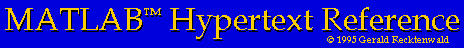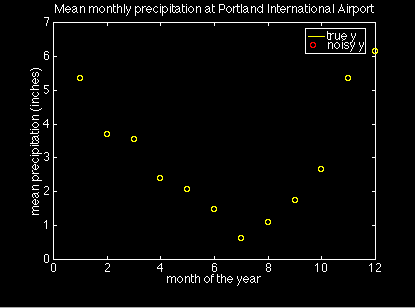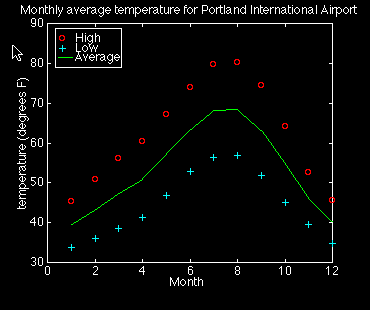# Loading Data into MATLAB for Plotting

In addition to plotting values created with its own commands, MATLAB is very useful for plotting data from other sources, e.g., experimental measurements. Typically this data is available as a plain text file organized into columns. MATLAB can easily handle tab or space-delimited text, but it cannot directly import files stored in the native (binary) format of other applications such as spreadsheets.

The simplest way to import your data into MATLAB is with the `load` command. Unfortunately, the `load` command requires that your data file contain no text headings or column labels. To get around this restriction you must use more advanced file I/O commands. Below I demonstrate both approaches with examples. I've included an m-file to handle the more complex case of a file with an arbitrary number of lines of text header, in addition to text labels for each column of data. Though hardly a cure-all, this function is much more flexible than the `load` command because it allows you to provide documentation inside your data file.

Here's an outline of this section

## The MATLAB `load` Command

There is more than one way to read data into MATLAB from a file. The simplest, though least flexible, procedure is to use the `load` command to read the entire contents of the file in a single step. The `load` command requires that the data in the file be organized into a rectangular array. No column titles are permitted. One useful form of the `load` command is

```            load name.ext
```

where ``name.ext'' is the name of the file containing the data. The result of this operation is that the data in ``name.ext'' is stored in the MATLAB matrix variable called `name`. The ``ext'' string is any three character extension, typically ``dat''. Any extension except ``mat'' indicates to MATLAB that the data is stored as plain ASCII text. A ``mat'' extension is reserved for MATLAB matrix files (see ``help load'' for more information).

Suppose you had a simple ASCII file named my_xy.dat that contained two columns of numbers. The following MATLAB statements will load this data into the matrix ``my_xy'', and then copy it into two vectors, x and y.

```	>> load my_xy.dat;     %  read data into the my_xy matrix
>> x = my_xy(:,1);     %  copy first column of my_xy into x
>> y = my_xy(:,2);     %  and second column into y
```

You don't need to copy the data into x and y, of course. Whenever the ``x'' data is needed you could refer to it as my_xy(:,1). Copying the data into ``x'' and ``y'' makes the code easier to read, and is more aesthetically appealing. The duplication of the data will not tax MATLAB's memory for most modest data sets.

The load command is demonstrated in the following example.

If the data you wish to load into MATLAB has heading information, e.g., text labels for the columns, you have the following options to deal with the heading text.

• Delete the heading information with a text editor and use the `load` command :-(

• Use the `fgetl` command to read the heading information one line at at time. You can then parse the column labels with the `strtok` command. This technique requires MATLAB version 4.2c or later.

• Use the `fscanf` command to read the heading information.

Of these options, using `fgetl` and `strtok` is probably the most robust and convenient. If you read the heading text into MATLAB, i.e., if you don't use the `load` command, then you will have to also read the plot data with `fscanf`. The example, Plotting data from files with column headings shows how this is done.

## A simple plot of data from a file

This example show you how to load a simple data set and plot it.

The PDXprecip.dat file contains two columns of numbers. The first is the number of the month, and the second is the mean precipitation recorded at the Portland International Airport between 1961 and 1990. (For an abundance of weather data like this check out the Oregon Climate Service)

Here are the MATLAB commands to create a symbol plot with the data from PDXprecip.dat. These commands are also in the script file precipPlot.m for you to download.

```	>> load PDXprecip.dat;         %  read data into PDXprecip matrix
>> month = PDXprecip(:,1);     %  copy first column of PDXprecip into month
>> precip = PDXprecip(:,2);    %  and second column into precip

>> plot(month,precip,'o');     %  plot precip vs. month with circles

>> xlabel('month of the year');           % add axis labels and plot title
>> ylabel('mean precipitation (inches)');
>> title('Mean monthly precipitation at Portland International Airport');
```

Although the data in the `month` vector is trivial, it is used here anyway for the purpose of exposition. The preceding statments create the following plot.## Plotting data from files with column headings

If all your data is stored in files that contain no text labels, the `load` command is all you need. I like labels, however, because they allow me to document and forget about the contents of a file. To use the `load` for such a file I would have to delete the carefully written comments everytime I wanted to make a plot. Then, in order to minimize my effort, I might stop adding the comments to the data file in the first place. For us control freaks, that leads to an unacceptable increase in entropy of the universe! The solution is to find a way to have MATLAB read and deal with the text comments at the top of the file.

The following example presents a MATLAB function that can read columns of data from a file when the file has an arbitrary length text header and text headings for each columns.

The data in the file PDXtemperature.dat is reproduced below

```	Monthly averaged temperature (1961-1990) Portland International Airport
Source:  Dr. George Taylor,
Oregon Climate Service, http://www.ocs.oregonstate.edu/

Temperatures are in degrees Farenheit

Month   High     Low    Average
1	45.36	33.84	39.6
2	50.87	35.98	43.43
3	56.05	38.55	47.3
4	60.49	41.36	50.92
5	67.17	46.92	57.05
6	73.82	52.8	63.31
7	79.72	56.43	68.07
8	80.14	56.79	68.47
9	74.54	51.83	63.18
10	64.08	44.95	54.52
11	52.66	39.54	46.1
12	45.59	34.75	40.17
```

The file has a five line header (including blank lines) and each column of numbers has a text label. To use this data with the `load` command you would have to delete the text labels and save the file. A better solution is to have MATLAB read the file without destroying the labels. Better yet, we should be able to tell MATLAB to read and use the column headings when it creates the plot legend.

There is no built-in MATLAB command to read this data, so we have to write an m-file to do the job. One solution is the file readColData.m. The full text of that function won't be reproduced here. You can click on the link to examine the code and save it on your computer if you like.

Here is the prologue to readColData.m

```	function  [labels,x,y] = readColData(fname,ncols,nhead,nlrows)
%  readColData  reads data from a file containing data in columns
%               that have text titles, and possibly other header text
%
%  Synopsis:
%     [labels,x,y] = readColData(fname)
%     [labels,x,y] = readColData(fname,ncols)
%     [labels,x,y] = readColData(fname,ncols,nhead)
%     [labels,x,y] = readColData(fname,ncols,nhead,nlrows)
%
%  Input:
%     fname  = name of the file containing the data (required)
%     ncols  = number of columns in the data file.  Default = 2.  A value
%              of ncols is required only if nlrows is also specified.
%     nhead  = number of lines of header information at the very top of
%              the file.  Header text is read and discarded.  Default = 0.
%              A value of nhead is required only if nlrows is also specified.
%     nlrows = number of rows of labels.  Default = 1
%
%  Output:
%     labels  =  matrix of labels.  Each row of lables is a different
%                label from the columns of data.  The number of columns
%                in the labels matrix equals the length of the longest
%                column heading in the data file.  More than one row of
%                labels is allowed.  In this case the second row of column
%                headings begins in row ncol+1 of labels.  The third row
%                column headings begins in row 2*ncol+1 of labels, etc.
%
%          NOTE:  Individual column headings must not contain blanks
%
%     x = column vector of x values
%     y = matrix of y values.  y has length(x) rows and ncols columns
```

The first line of the file is the function definition. Following that are several lines of comment statements that form a prologue to the function. Because the first line after the function definition has a non-blank comment statement, typing ``help readColData'' at the MATLAB prompt will cause MATLAB to print the prologue in the command window. This is how the on-line help to all MATLAB functions is provided.

The prologue is organized into four sections. First is a brief statement of what the function does. Next is a synopsis of the ways in which the function can be called. Following that the input and output parameters are described.

Here are the MATLAB commands that use readColData.m to plot the data in PDXtemperature.dat. The commands are also in the script file multicolPlot.m

```	>>  %  read labels and x-y data
>>  [labels,month,t] = readColData('PDXtemperature.dat',4,5);

>>  plot(month,t(:,1),'ro',month,t(:,2),'c+',month,t(:,3),'g-');

>>  xlabel(labels(1,:));                      % add axis labels and plot title
>>  ylabel('temperature (degrees F)');
>>  title('Monthly average temperature for Portland International Airport');

>>  %  add a plot legend using labels read from the file
>>  legend(labels(2,:),labels(3,:),labels(4,:));
```

These statments create the following plot.